Next: 3.2.2 Energy Relaxation Time Up: 3.2 Physical Parameters Previous: 3.2 Physical Parameters

## 3.2.1 Mobility Model

The mobility model used has proven its usefulness during the last decades . The temperature dependence of the lattice mobility in Si is modeled by a power law.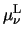=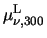.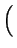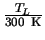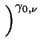,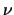= n, p (3.21)

To account for mobility reduction due to ionized impurity scattering, the formula of Caughey and Thomas  is used in conjunction with temperature dependent coefficients. CI denotes the concentration of ionized impurities.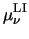=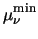+(3.22)=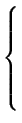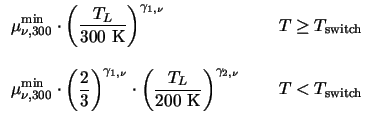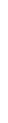(3.23) Cref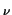= Cref, 300 .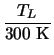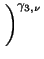(3.24)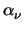=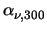.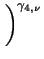(3.25)

Surface scattering is modeled by the following empirical expression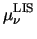=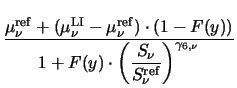(3.26)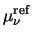=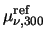.(3.27) F(y) =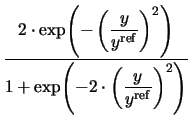(3.28)

The pressing forces Sn and Sp in (3.26) are equal to the magnitude of the normal field strength at the interface if the carriers are attracted by the interface, otherwise zero.

For the DD transport model mobility reduction due to a high-field is modeled by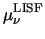=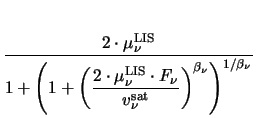(3.29) F=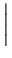grad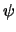+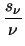.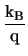. grad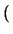TL .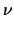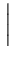(3.30)

with Frepresenting the driving forces for carrier.

For the HD transport model deviation from the ohmic low-field mobility is modeled as a function of the carrier temperature, Tafter Hänsch .=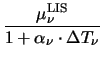(3.31)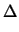T= T- TL (3.32)=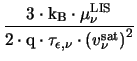(3.33)

where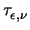denotes the energy relaxation time.Next: 3.2.2 Energy Relaxation Time Up: 3.2 Physical Parameters Previous: 3.2 Physical Parameters
Tibor Grasser
1999-05-31